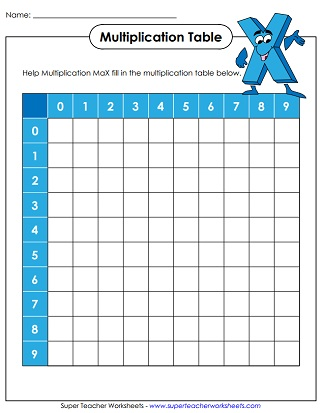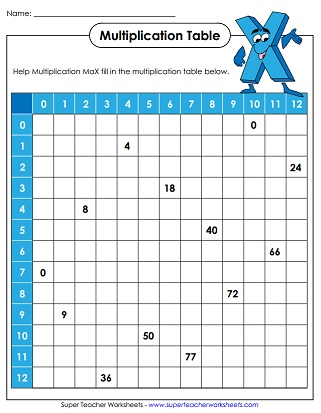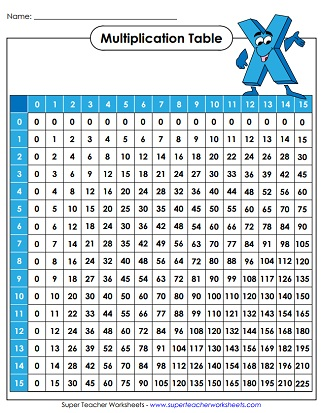# Multiplication Tables (Multiplication Charts)

For Basic Fact Worksheets, please go to this section of the site: Multiplication Worksheets

For worksheets on multiplying bigger numbers, then you'll want to go here: Double Digit Multiplication.## Multiplication 0-9

This entire table is filled in so  students can use it for reference purposes.
Fill in the products on these mini-multiplication tables. This page has illustrations of children playing with cars and trains.
Complete the mini-multiplication tables by writing the missing factors and products. This worksheet has pirate-themed graphics.
This multiplication table is only partly filled in.  Students can fill in the empty squares.
This zero through nine multiplication table is blank, so students can fill in the answers.
This page contains four miniature desktop charts that you can cut out for student reference.

## Multiplication 0-12

All of the boxes are filled in; Use as a reference chart.
Many of the boxes are empty, but some are already filled in.
Here's a blank 0 through 12 multiplication table has empty boxes for students to fill in.
Here's a set of four miniature reference tables that can be cut apart.
Print out this empty multiplication fact sheet that ranges from 0 through 12.
Print out this filled in multiplication fact sheet that ranges from 0 through 12.

## Multiplication 0-15

Fill in an empty multiplication table, from 0-15.
A partially filled in multiplication table, from 0-15.
Filled in multiplication table from 0-15.
Print this set of four miniature 0-15 multiplication tables for students' desktops.

## Multiplication Arrays

Cut and use the L-shaped tool to view an array for any basic multiplication fact up to 10.

## Integers

This multiplication table includes negative numbers. It has facts from -4 up to +4.

Multiplication Fact Worksheets & Games

Lattice Multiplication Worksheets & Grids

Blank lattice grids and printable lattice problems for practice.

Properties of Multiplication

The worksheets in this section focus on the properties of multiplication, including the associative property and the distributive property.

## Pictures of Our WorksheetsMy Account
Site Information